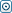Forex Stock Exchange Forum
Would you like to react to this message? Create an account in a few clicks or log in to continue.

# Statistical evaluation for successful investmentH.W.FX
Number of messages : 19
Points : 2235
Date of Entry : 2015-08-21
Year : 41

##Statistical evaluation for successful investment

Fri Aug 21, 2015 6:50 pm
Statistical evaluation for successful investment, which is based on a search of the quantile
Recommended timeframes D1

Quantile – is the value of the distribution function (Gaussian function), the value of this function shall not exceed or go below the given value with a certain probability.
In our example we will analyze the currency pair EUR/USD. In order to calculate the quantile, we shall review the history of the quotes; the data shall include at least 250 examples.
We go to: Trading terminal – Tools -> data store of the quotes -> Forex Major -> EUR/USD-> Day -> Export.
Then we put the derived data into EXCEL table in order to make calculations.
Once we put the data into EXCEL table, we will calculate the yield using the formula: LN(C2/C3)

[You must be registered and logged in to see this image.]

After that we shall calculate expected value and standard deviation, using the standard formulas in EXCEL:
Expected value = (D2:D249)
Standard deviation = (D2:D249)
[You must be registered and logged in to see this image.]

After the expected value and standard deviation is known, we can calculate Quantile using EXCELL formula
Quantile =(1%;E2;F2)
[You must be registered and logged in to see this image.]

In order to calculate the exchange rate for the next day within the accuracy of 99%, you shall multiply the existing price of the pair EUR/USD by quantile + one.

Xt+1 = (Q+1)* Xt;

Where: Q- the value of quantile for the normal distribution of EUR/USD;
Xt- the value of the latest quote at this point of time;
Xt+1 – the value of the exchange rate for the next point of time

Calculations are made in EXCEL as follows:
Xt+1 =(1+G2)*C249 – calculations for the next day

[You must be registered and logged in to see this image.]

Xt+n = (Q+1* √n)* Xt;

Where: Q- the value of quantile for the normal distribution of EUR/USD;

Xt- the value of the latest quote at this point of time;

Xt+n – the value of the exchange rate for a few coming days

N –the number of the coming days

Xt+n =(SQRT(5)*G2+1)*C249

[You must be registered and logged in to see this image.]

As a result we have:

X1 = 1.115542939 – shows a probability of 99% that on the next day the rate will not fall below this value.

X2= 1.093099577 – shows a probability of 99% that the rate will not drop below this value in the next 5 days

Full calculations are provided in EXCEL. This analysis can be applied for all financial instruments. It is important to remember that the more examples (historical data) we use, the more accurate statistics we will get.

Permissions in this forum:
You cannot reply to topics in this forum